# Computing Thermal Resistance of PCB-Mounted MMIC Devices

## Computing Thermal Resistance of PCB-Mounted MMIC Devices

Temperature has a direct impact on the operating performance and reliability of semiconductor devices. Devices that operate at high junction temperatures for long periods of time can sustain permanent damage, compromising reliability and shortening operating lifetimes.  The life of a MMIC component is directly related to the temperature at the hot spot of the semiconductor die in the device.  It is therefore critical that designers understand the thermal properties of a PCB design for a given MMIC device in order to minimize thermal resistance and heat rise.

This article discusses a general method for computing thermal resistance between Mini-Circuits MMIC amplifier model PHA-1+ and ambient.  Junction temperature will be calculated based on thermal resistance of the printed circuit board (PCB) layout and measured using thermal photography.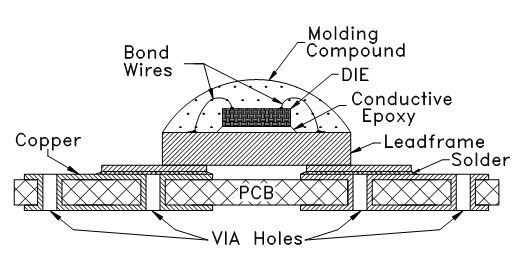Figure 1: Cross section of a MMIC component

A packaged MMIC component consists of a semiconductor die mounted on a lead frame as shown in figure 1.  The die is attached to the lead frame using conductive epoxy or solder, and electrical connections from the die to the lead frame are made using wire bonding.  The die is covered with a plastic molding compound to protect it from environmental damage and contamination.

Thermal resistance is defined as the ratio of temperature rise to the power dissipated and is expressed in °C/W.  The thermal resistance from the hot spot on the die to external ambient consists of a series of thermal resistances:

• Hotspot on the die to bottom of die (θdie )
• Conductive epoxy (θce )
• Solder on PCB (θsl )
• Top of PCB to bottom surface (θpCB )
• PCB to ambient (θpCB-A )

Out of the six factors, the sum of thermal resistances of the die, conductive epoxy and lead frame is usually called θjc, or thermal resistance from junction to case, which is typically provided by component manufacturers.  For PHA-1+ this is 60°C/W. The contribution of solder on the PCB (θsl) is small and not discussed in this article. What remains to be determined is the thermal resistance of the PCB.

Take Mini-Circuits PHA-1+ high-dynamic range MMIC amplifier as an example.  The amplifier operates on a single +5V power supply and draws 165 mA and is housed in an industry standard SOT-89 package.  The suggested PCB layout for this device is shown in figure 1.  The layout must accommodate both the electrical requirements of the device (such as proper line impedance, bypassing, etc.) and the thermal requirements.  Via holes in the PCB play an important part in providing good electrical and thermal paths to ground.  The recommended PCB layout for PHA-1+ consists of 7 via holes under the amplifier unit.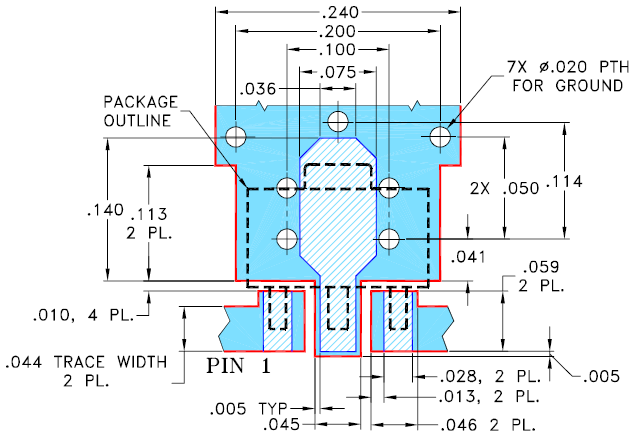Figure 2: Suggested PCB layout of Mini-Circuits’ PHA-1+ MMIC amplifier

The thermal resistance (θ) of a single via hole can be calculated using equation 1: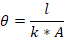(Eq.1)

Where:
l= length (m)
A= Area (m2)
k= Thermal conductivity (W/m-K)

Referring to equation 1, for an unfilled via: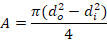(Eq. 2)

Where:
d_o= Outer diameter of the via (m)
d_i= Inner diameter of the via (m)
Note that the difference between d_o and d_i is the copper thickness.

Substituting Equation 2 into Equation 1, the thermal resistance of a single copper via is expressed as: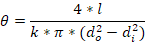(Eq. 3)

Equation 3 implies that thermal resistance, θ will decrease as:

• Length of the via decreases
• Via diameter (increases
• Copper thickness in the via increases

For PHA-1+, each of the 7 via holes in the suggested PCB layout has a 0.02” outer diameter (d_o) with a copper thickness of 0.0014”, resulting in an inner diameter (d_i) of 0.0172”.  PCB thickness is 0.020”.  Also given 0.0014” copper thickness on the top and bottom of the PCB, the length of the via (l) is 0.0228”.

Substituting these parameters into Equation 3, the thermal resistance of a 20-mil diameter unfilled via hole is 25˚C/W.  For 7 via holes, we assume the thermal resistance is calculated as if they were in parallel to simplify the calculation.  Thermal resistance for 7 vias in parallel is 4.1˚C/W.  This method does not take into account the spatial distribution of the vias, but it provides a good first order approximation.

Given that thermal resistance is the ratio of temperature rise to power dissipated, the value for thermal resistance allows us to predict the temperature rise as: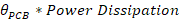(Eq.4)

Where Power Dissipation is the product of device voltage and device current.  For a PHA-1+ amplifier, device voltage is 5V and operating current is 0.165W, so power dissipation is 0.825W.  Temperature rise is therefore:

4.1°C/W x 0.825W = 3.4°C

This is a first order approximation of the temperature rise from the device case to the ground lug.

***

To validate the accuracy of this finding, 5V supply was applied to the DC terminal of the test circuit for PHA-1+ (TB-545-1+), shown in figure 3, with measured DC current of 165 mA.  After temperature stabilized, a thermal photograph was taken to measure temperature of the case, PCB, ground lug and PHA-1+ body as shown in figure 4.  The measurement results are shown and compared to the calculations above in Table 1.Figure 3: Test board for PHA-1+ (TB-545-1+)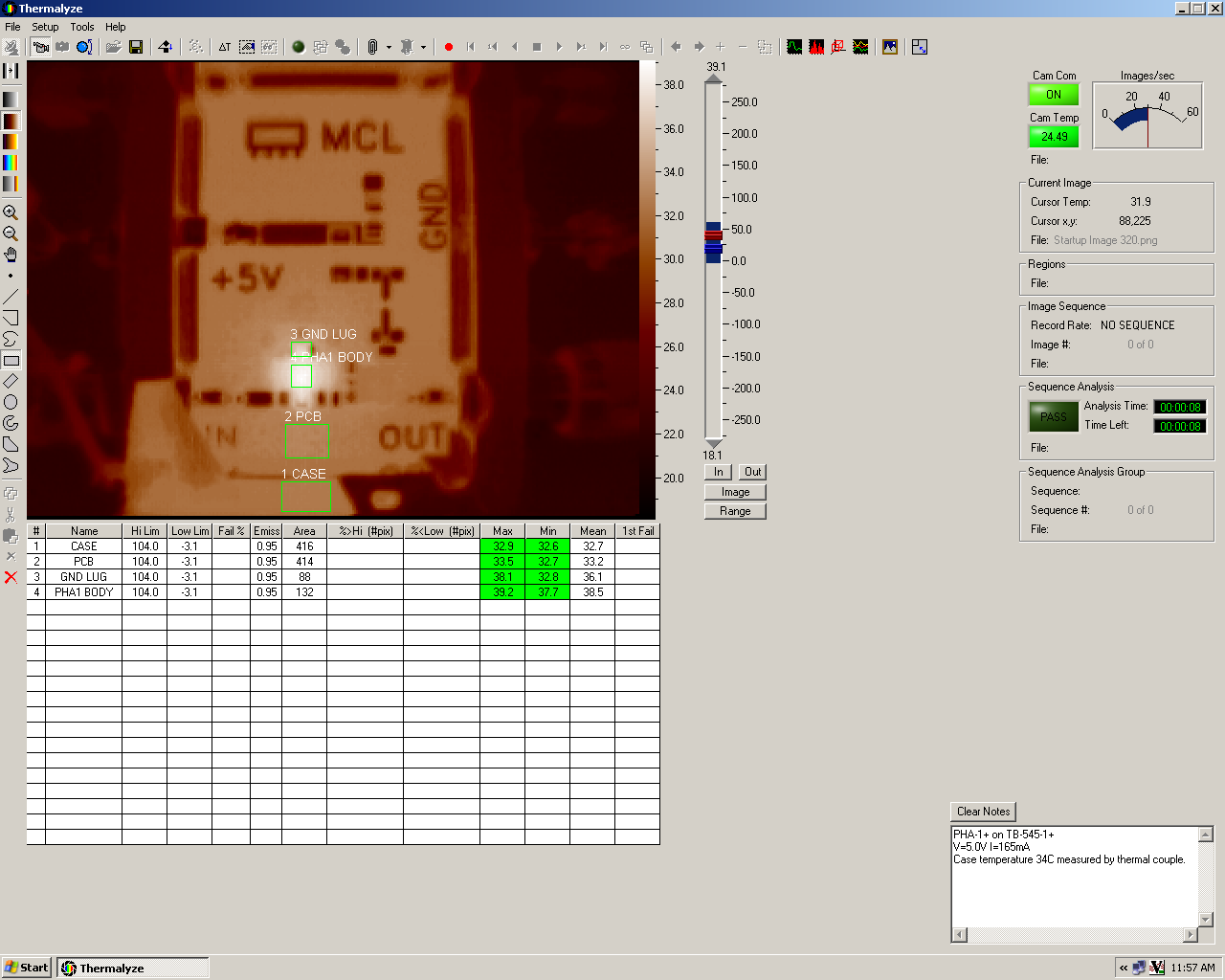Figure 4: Thermal image of PHA-1+ to measure temperature of case, PCB, ground lug and PHA-1+ body

 Computed (deg C) Measured (deg C_) Difference ϴvia x Pd Case GND Rise 3.4 32.7 36.1 3.4 0.0

Table 1: Computed temperature rise versus measured temperature rise

The measured temperature (mean) of the case (assuming it is same as the bottom of the PCB) was 32.7°C; the measured temperature (mean) of the ground lug was 36.1°C.  Therefore the measured temperature rise from case to ground lug was 3.4°C, which is same as the computed value.  The method for calculating thermal resistance shown in this article therefore allows designers to accurately predict temperature rise without the need for sophisticated thermal analysis tools and software.  This is a useful tool in designing a PCB to minimize thermal resistance.

The calculation demonstrated above allows accurate prediction of thermal resistance from PCB to case.  Often, thermal resistance from junction to ambient is needed.  In the case of PHA-1+, given an ambient temperature of 24°C and measured case temperature of 32.7°C, the difference between case and ambient temperature is 8.4°C

Therefore, Thermal resistance from case to ambient is: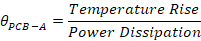= 8.4°C / (5V * 0.165mA) = 10.5°C/W

Therefore total thermal resistance from junction to ambient is: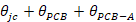= 60°C + 4.1°C + 10.5°C

= 74.6 °C/W

<< קרא עוד

<< קרא עוד

<< קרא עוד